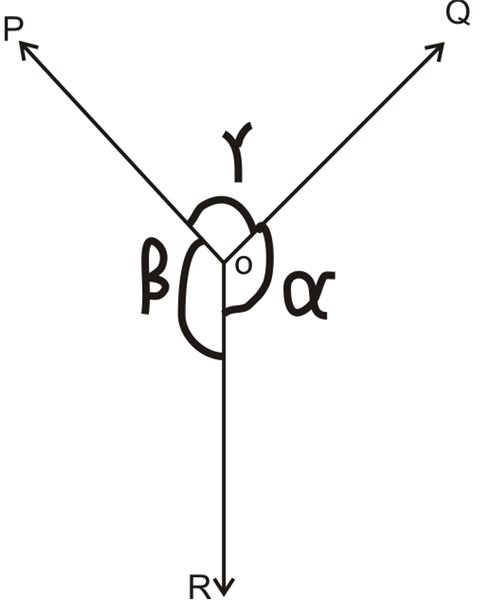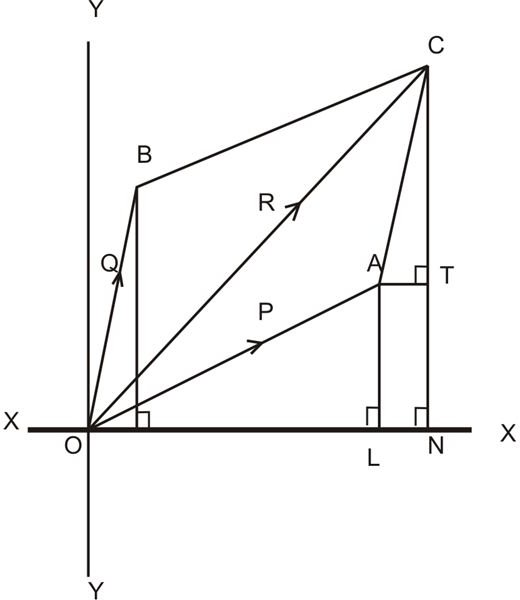# Karann## Calculating Equilibrium of Forces Through Lami’s Theorem

The article explains the conditions required for a set of forces acting over a body to come under equilibrium, during which the body remains stationary even under the influence of the forces. We will also discuss methods for calculating this phenomenon using Lami’s theorem.## What is Force Resolution, Polygon Law of Forces, and Triangle Law of Forces?

Force may be defined as an agent which generates or has a tendency to generate a motion and in some occasions “kills” or “tends to kill” a motion. Here, we investigate more about force and methods of calculating the resultant of a group of forces acting over a common particle.